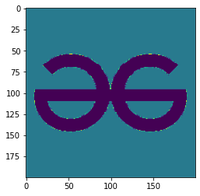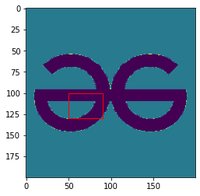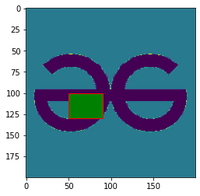GFG App
Open AppBrowser
Continue

# How to Draw Rectangle on Image in Matplotlib?

Prerequisites: Matplotlib

Given an image the task here is to draft a python program using matplotlib to draw a rectangle on it. Matplotlib comes handy with rectangle() function which can be used for our requirement.

Syntax: Rectangle(xy, width, height, angle=0.0, **kwargs)

Parameters:

• xy: Lower left point to start the rectangle plotting
• width : width of the rectangle
• height: Height of the rectangle.
• angle: Angle of rotation of the rectangle.

### Approach

• Import the necessary libraries.
• Insert and display the image.
• Create the figure and axes of the plot
• Add the patch to the Axes
• Display the image

Example 1: Draw a rectangle on an image

## Python3

 `import` `matplotlib.pyplot as plt ` `import` `matplotlib.patches as patches ` `from` `PIL ``import` `Image ` `import` `numpy as np ` ` `  `x ``=` `np.array(Image.``open``(``'geek.png'``), dtype``=``np.uint8) ` `plt.imshow(x) ` ` `  `# Create figure and axes ` `fig, ax ``=` `plt.subplots(``1``) ` ` `  `# Display the image ` `ax.imshow(x) ` ` `  `# Create a Rectangle patch ` `rect ``=` `patches.Rectangle((``50``, ``100``), ``40``, ``30``, linewidth``=``1``, ` `                         ``edgecolor``=``'r'``, facecolor``=``"none"``) ` ` `  `# Add the patch to the Axes ` `ax.add_patch(rect) ` `plt.show() `

Output:original imageimage with rectangle

Example 2: Draw a filled rectangle

## Python3

 `import` `matplotlib.pyplot as plt ` `import` `matplotlib.patches as patches ` `from` `PIL ``import` `Image ` `import` `numpy as np ` ` `  `x ``=` `np.array(Image.``open``(``'geek.png'``), dtype``=``np.uint8) ` `plt.imshow(x) ` ` `  `# Create figure and axes ` `fig, ax ``=` `plt.subplots(``1``) ` ` `  `# Display the image ` `ax.imshow(x) ` ` `  `# Create a Rectangle patch ` `rect ``=` `patches.Rectangle((``50``, ``100``), ``40``, ``30``, linewidth``=``1``, ` `                         ``edgecolor``=``'r'``, facecolor``=``"g"``) ` ` `  `# Add the patch to the Axes ` `ax.add_patch(rect) ` `plt.show() `

Output:original imageimage with rectangle

My Personal Notes arrow_drop_up